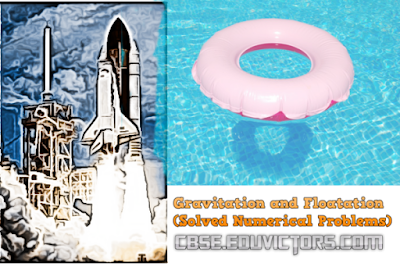## Pages

Showing posts with label class9-physics. Show all posts
Showing posts with label class9-physics. Show all posts

# Physics: Important Discoveries

No. Discovery Scientist Year
1. Proton Rutherford 1919
3. Atom John Dalton 1808
4. Atomic Structure Neil Bohr & Rutherford 1913
7. Raman Effect CV. Raman 1928
8. X-Rays Roentgen 1895
9. Photoelectric effect Albert Einstein 1905
10. Periodic table Mendeleev 1888
11. Nuclear Reactor Anrico Fermi 1942
12. Wireless Telegram Marconi 1901
13. Dynamite Alfred Nobel 1867## Monday, 31 December 2018

### CBSE Class 9 - Physics - Work, Power and Energy - Worksheet (#eduvictors)(#cbsenotes)

Class 9 - Physics

## Work, Power and Energy

Worksheet

Fill in the blanks with suitable words.

1. Energy is the __________ to do work. Its S.I. unit is ________.

2. Work is force multiplied by ___________ in the direction of force.

3. If the angle between force and the displacement caused is acute then work is said to be ________.

4. If the angle between force and the displacement caused is obtuse, the work is said to be _______.

5. One joule of work is said to be done when a force of ______ displaces a body through a distance of ________ in its own direction.## CBSE Class 9 - Physics -Gravitation and Flotation (Solved Numerical Problems)

Questions:

1) Find the total thrust acting on the bottom surface of a tank 4m long, 2m broad and 2m deep when fully filled with water. The density of water= 10³ kg/m³.

2) The gravitational force between two objects is 100N. How should the distance between the objects be changed so that force between them becomes 50N?

3) If the distance between two masses be increased by a factor of 6, by what factor would the mass of one of them have to be altered to maintain the same gravitational force? Would this be an increase or decrease in the mass?

4) The value of ‘g’ on earth’s surface is 9.8 m/s². Suppose the earth suddenly shrinks to one-third of its present size without losing any mass. What is the value of ‘g’ on the surface of shrunk earth?

5) Two bodies of mass 1kg and 100kg respectively are dropped simultaneously from same height 4.9m in a vacuum. Calculate and compare their final velocities just before hitting the ground and the time interval in which they will hit the ground.

6) The ratio of gravitational force on Neptune to the gravitational force on earth is 9:8. Ramesh weighs 792N on earth. Calculate his weight on Neptune.